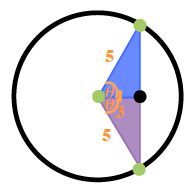### Home > PC3 > Chapter 2 > Lesson 2.2.5 > Problem2-118

2-118.

Given: $\cos(θ)=\frac{3}{5}$

1. Draw a right triangle and place values on the sides so that $\cos(θ)=\frac{3}{5}$.2. Draw a unit circle diagram to clearly show the two possible positions for $θ$.3. Use the Pythagorean Identity or a right triangle to solve for all possible values for $\sin(θ)$.

It must be a 3-4-5 triangle because of the Pythagorean Theorem. Determine the two sine values.

4. Solve for all possible values for $\tan(θ)$.

$\operatorname { tan }(\theta)=\frac {\text{opposite}}{\text{adjacent}}=\frac{\pm4}{3}=\pm\frac{4}{3}$